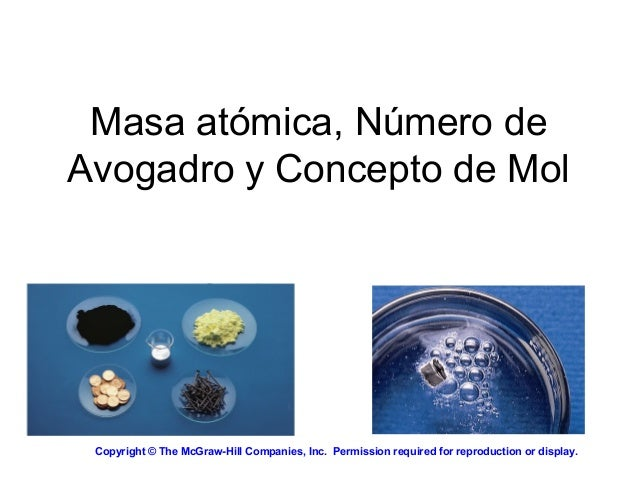Successfully reported this slideshow.
We use your LinkedIn profile and activity data to personalize ads and to show you more relevant ads. You can change your ad preferences anytime.Upcoming SlideShare
Loading in …5
×

# MASA ATOMICA, MASA MOLAR Y NUMERO DE AVOGADRO

Presentación orientada al esclarecimiento de los conceptos de masa atómica y molar de los estudiantes de Nivel Introductorio de la Sede UIS Barbosa.

• Full Name
Comment goes here.

Are you sure you want to Yes No
Your message goes here• Be the first to comment

### MASA ATOMICA, MASA MOLAR Y NUMERO DE AVOGADRO

1. 1. Masa atómica, Número de Avogadro y Concepto de Mol Copyright © The McGraw-Hill Companies, Inc.  Permission required for reproduction or display.
2. 2. Copyright © The McGraw-Hill Companies, Inc.  Permission required for reproduction or display. Esta presentación ha sido diseñada por los creativos de Mc Graw Hill Companies INC. La docente Raquel Villafrades Torres modificó algunos elementos de forma para facilitar la comprensión de los aspectos aquí plasmados. Su uso tiene fines educativos como el favorecimiento del proceso de aprendizaje de los estudiantes de Nivel Introductorio en la Sede UIS Barbosa. ACLARACIÓN IMPORTANTE:
3. 3. Por definición: 1 átomo 12 C “pesa” 12 uma En esta escala: 1 H = 1.008 uma 16 O = 16.00 uma La masa atómica es la masa de un átomo en unidades de masa atómica (uma) Micro-mundo Átomos y moléculas Macro-mundo gramos
4. 4. El litio en la naturaleza se encuentra como (isótopos): 7.42% 6 Li (6.015 uma) 92.58% 7 Li (7.016 uma) 7.42 x 6.015 + 92.58 x 7.016 100 = 6.941 uma Masa atómica promedio del litio:
5. 5. Masa atómica promedio (6.941)
6. 6. Un mol es la cantidad de sustancia que contiene tantos átomos como hay en exactamente 12.00 gramos de 12 C. 1 mol = NA = 6.0221367 x 1023 El número de Avogadro (NA) DOCENA = 12 PAR = 2
7. 7. La masa molar es la masa molecular expresada en gramos 1 mol de átomos 12 C es = 6.022 x 1023 átomos = 12.00 g 1 átomo 12 C = 12.00 uma 1 mol de átomos 12 C = 12.00 g 12 C 1 mol de átomos de litio = 6.941 g de Li Para cualquier elemento masa atómica (uma) = masa molar (gramos)
8. 8. Un mol de: C S Cu Fe Hg 3.2
9. 9. 1 uma = 1.66 x 10-24 g o 1 g = 6.022 x 1023 uma 1 12 C átomo 12.00 uma x 12.00 g 6.022 x 1023 12 C átomos = 1.66 x 10-24 g 1 uma M = masa molar en g/mol NA = Número de Avogadro (partículas/mol)
10. 10. x 6.022 x 1023 átomos K 1 mol K = ¿Entiendes qué es la masa molar? ¿Cuántos átomos hay en 0.551 g de potasio (K) ? 1 mol K = 39.10 g K 1 mol K = 6.022 x 1023 átomos K 0.551 g K 1 mol K 39.10 g K x 8.49 x 1021 átomos K
11. 11. Masa molecular (No peso molecular) es la suma de masas atómicas (en uma) de los elementos de una molécula. SO2 1S 32.07 uma 2O + 2 x 16.00 uma SO2 64.07 uma Para cualquier molécula masa molecular (uma) = masa molar (gramos) 1 molécula SO2 = 64.07 uma 1 mol SO2 = 64.07 g SO2
12. 12. ¿Entiendes que es la masa molecular? ¿Cuántos átomos de H hay en 72.5 g of C3H8O ? 1 mol C3H8O = (3 x 12) + (8 x 1) + 16 = 60 g C3H8O 1 mol- átomos H = 6.022 x 1023 átomos H 5.82 x 1024 átomos de H 1 mol - moléculas C3H8O = 8 mol átomos de H 72.5 g C3H8O 1 mol C3H8O 60 g C3H8O x 8 mol átomos H 1 mol C3H8O x 6.022 x 1023 átomos H 1 mol átomos H x =
13. 13. La masa formular es la suma de las masas atómicas (en uma) en una fórmula unitaria de un compuesto iónico. 1Na 22.99 uma 1Cl + 35.45 uma NaCl 58.44 uma Para cualquier compuesto iónico masa de la fórmula (uma) = masa molar (gramos) 1 fórmula unitaria NaCl = 58.44 uma 1 mol NaCl = 58.44 g NaCl NaCl
14. 14. ¿Entiendes qué es la masa formular? ¿Cuál es la masa formular de Ca3(PO4)2 ? 1 fórmula unitaria de Ca3(PO4)2 3 Ca 3 x 40.08 2 P 2 x 30.97 8 O + 8 x 16.00 310.18 uma
15. 15. Ek = 1/2 x m x v2 v = (2 x Ek/m)1/2 F = q x v x B Ligero Ligero Pesado Pesado
16. 16. Composición porcentual de un elemento en un compuesto = n x masa molar del elemento masa molar del compuesto x 100% n es el número de mol - átomos del elemento en 1 mol del compuesto C2H6O %C = 2 x (12.01 g) 46.07 g x 100% = 52.14% %H = 6 x (1.008 g) 46.07 g x 100% = 13.13% %O = 1 x (16.00 g) 46.07 g x 100% = 34.73% 52.14% + 13.13% + 34.73% = 100.0%
17. 17. Composición porcentual y fórmulas empíricas Determine la fórmula de un compuesto que tiene la siguiente composición porcentual en peso: 24.75 % K, 34.77 % Mn, 40.51 % O nK = 24.75 g K x = 0.6330 mol K 1 mol K 39.10 g K nMn = 34.77 g Mn x = 0.6329 mol Mn 1 mol Mn 54.94 g Mn nO = 40.51 g O x = 2.532 mol O 1 mol O 16.00 g O
18. 18. K : ~~ 1.0 0.6330 0.6329 Mn : 0.6329 0.6329 = 1.0 O : ~~ 4.0 2.532 0.6329 nK = 0.6330, nMn = 0.6329, nO = 2.532 KMnO4 Composición porcentual y fórmulas empíricas